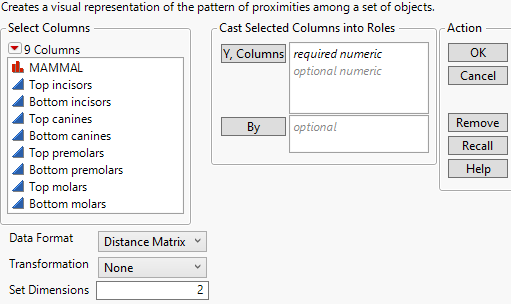Multivariate Methods > Multidimensional Scaling > Launch the Multidimensional Scaling Platform
Publication date: 08/13/2020

# Launch the Multidimensional Scaling Platform

Launch the Multidimensional Scaling Platform by selecting Analyze > Multivariate Methods > Multidimensional Scaling.

Figure 10.4 Multidimensional Scaling Launch WindowY, Columns

The columns to be analyzed. These must have a Numeric data type.

By

A column or columns whose levels define separate analyses. For each level of the specified column, the corresponding rows are analyzed using the other variables that you have specified. The results are presented in separate reports. If more than one By variable is assigned, a separate report is produced for each possible combination of the levels of the By variables.

Note: When using a distance matrix, the By variable requires a full matrix for each level of the By variable.

Data Format

MDS supports two data formats:

Distance Matrix

A full symmetric, lower, or upper triangular matrix where the number of rows equals the number of columns.The diagonal entries can either be zeros or missing.

Attribute List

A set of columns that contain measures of a quality or characteristic of an object. The objects are typically named in a column. The object column is not used in the analysis but rather is used as a label for the data points on the MDS plot.

Transformation

Supported transformations are Ratio, Interval, and Ordinal.

None

No transformation used.

Ratio

Data has an ordering from smallest to largest, the differences between values have meaning, and the scale has a true zero. Used to scale the MDS plot.

Interval

Data has an ordering from smallest to largest and the differences between values have meaning. Used to scale and shift the MDS plot.

Ordinal

Data has an ordering from smallest to largest. Used for ordinal data.

Set Dimensions

The number of dimensions for the visual representation of the proximities among your objects. Typically, two or three dimensions are used. With greater than three dimensions, the visualization becomes complex.

Note: The dimension selected can be between 1 to n - 1 where n = the number of objects, otherwise the dimension is set to 2.